Balbharti Maharashtra State Board Class 11 Maths Solutions Pdf Chapter 4 Determinants and Matrices Ex 4.6 Questions and Answers.

## Maharashtra State Board 11th Maths Solutions Chapter 4 Determinants and Matrices Ex 4.6

Question 1.
Evaluate:
i. $$\left[\begin{array}{l} 3 \\ 2 \\ 1 \end{array}\right]\left[\begin{array}{lll} {[2} & -4 & 3 \end{array}\right]$$
ii. $$\left[\begin{array}{lll} 2 & -1 & 3 \end{array}\right]\left[\begin{array}{l} 4 \\ 3 \\ 1 \end{array}\right]$$
Solution:
i. \begin{aligned} \left[\begin{array}{l} 3 \\ 2 \\ 1 \end{array}\right]\left[\begin{array}{lll} 2 & -4 & 3 \end{array}\right] &=\left[\begin{array}{lll} 3(2) & 3(-4) & 3(3) \\ 2(2) & 2(-4) & 2(3) \\ 1(2) & 1(-4) & 1(3) \end{array}\right] \\ &=\left[\begin{array}{ccc} 6 & -12 & 9 \\ 4 & -8 & 6 \\ 2 & -4 & 3 \end{array}\right] \end{aligned}

ii. $$\left[\begin{array}{lll} 2 & -1 & 3 \end{array}\right]\left[\begin{array}{l} 4 \\ 3 \\ 1 \end{array}\right]$$
= [2(4)-1(3)+ 3(1)]
= [8 – 3 + 3] =Question 2.
If A = $$\left[\begin{array}{cc} 1 & -3 \\ 4 & 2 \end{array}\right]$$ B = $$\left[\begin{array}{cc} 4 & 1 \\ 3 & -2 \end{array}\right]$$, = show that AB ≠ BA.
Solution: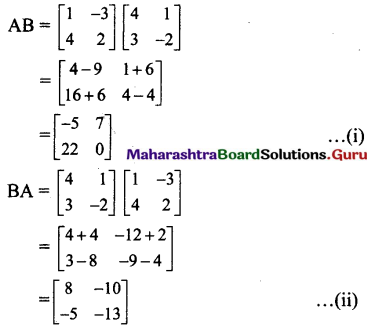From (i) and (ii), we get
AB ≠ BA

Question 3.
If A = $$\left[\begin{array}{ccc} -1 & 1 & 1 \\ 2 & 3 & 0 \\ 1 & -3 & 1 \end{array}\right]$$ ,B = $$\left[\begin{array}{lll} 2 & 1 & 4 \\ 3 & 0 & 2 \\ 1 & 2 & 1 \end{array}\right]$$ state whether AB = BA? Justify your answer.
Solution: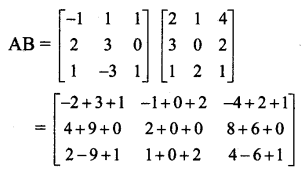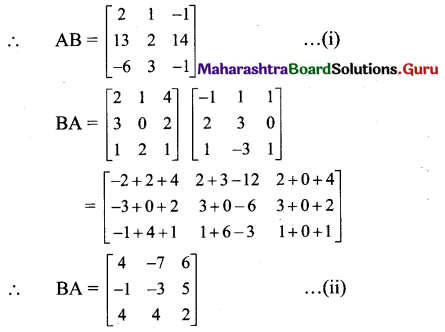From (i) and (ii), we get
AB ≠ BA

Question 4.
Show that AB = BA, where
i. A = $$\left[\begin{array}{rrr} -2 & 3 & -1 \\ -1 & 2 & -1 \\ -6 & 9 & -4 \end{array}\right]$$ , B = $$\left[\begin{array}{rrr} 1 & 3 & -1 \\ 2 & 2 & -1 \\ 3 & 0 & -1 \end{array}\right]$$
ii. A = $$\left[\begin{array}{cc} \cos \theta & \sin \theta \\ -\sin \theta & \cos \theta \end{array}\right]$$, B = $$\left[\begin{array}{cc} \cos \theta & -\sin \theta \\ \sin \theta & \cos \theta \end{array}\right]$$
Solution: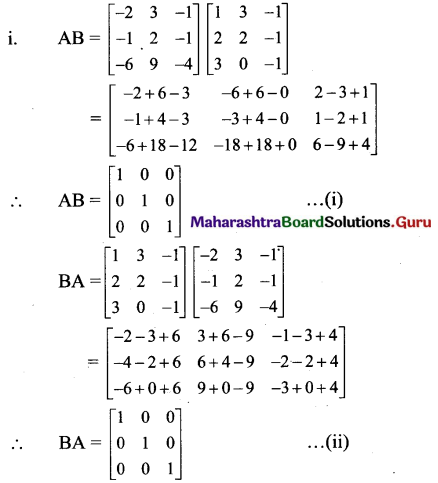From (i) and (ii), we get
AB = BA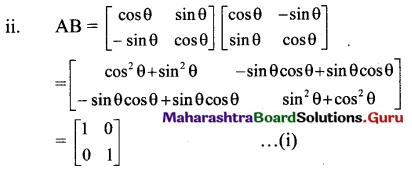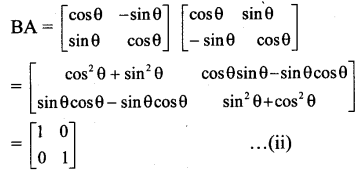From (i) and (ii), we get
AB = BA
[Note: The question has been modified.]Question 5.
If A = $$\left[\begin{array}{cc} 4 & 8 \\ -2 & -4 \end{array}\right]$$, prove that A2 = 0.
Solution:
A2 = A.A
= $$\left[\begin{array}{cc} 4 & 8 \\ -2 & -4 \end{array}\right]\left[\begin{array}{cc} 4 & 8 \\ -2 & -4 \end{array}\right]$$
= $$\left[\begin{array}{cc} 16-16 & 32-32 \\ -8+8 & -16+16 \end{array}\right]$$
= $$\left[\begin{array}{ll} 0 & 0 \\ 0 & 0 \end{array}\right]$$ = 0

Question 6.
Verify A(BC) = (AB)C in each of the following cases:
i. A = $$=\left[\begin{array}{cc} 4 & -2 \\ 2 & 3 \end{array}\right]$$, B = $$\left[\begin{array}{cc} -1 & 1 \\ 3 & -2 \end{array}\right]$$ and C = $$\left[\begin{array}{cc} 4 & 1 \\ 2 & -1 \end{array}\right]$$
ii. A = $$\left[\begin{array}{ccc} 1 & -1 & 3 \\ 2 & 3 & 2 \end{array}\right]$$, B = $$\left[\begin{array}{cc} 1 & 0 \\ -2 & 3 \\ 4 & 3 \end{array}\right]$$ and C = $$\left[\begin{array}{cc} 1 & 2 \\ -2 & 0 \\ 4 & -3 \end{array}\right]$$
Solution: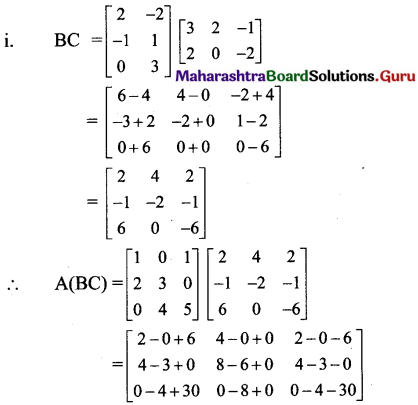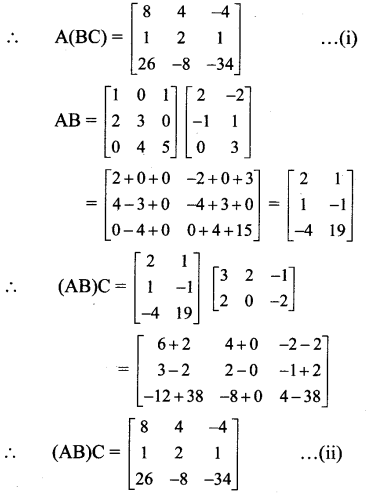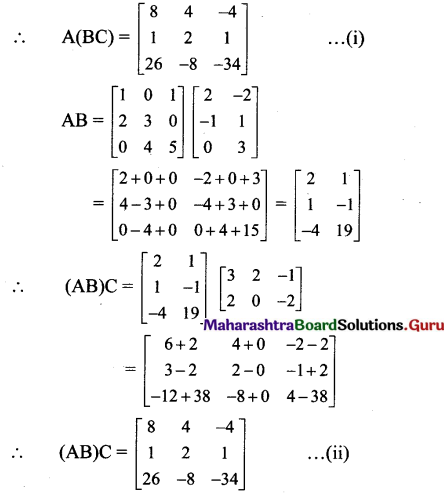From (i) and (ii), we get
A(BC) = (AB)C.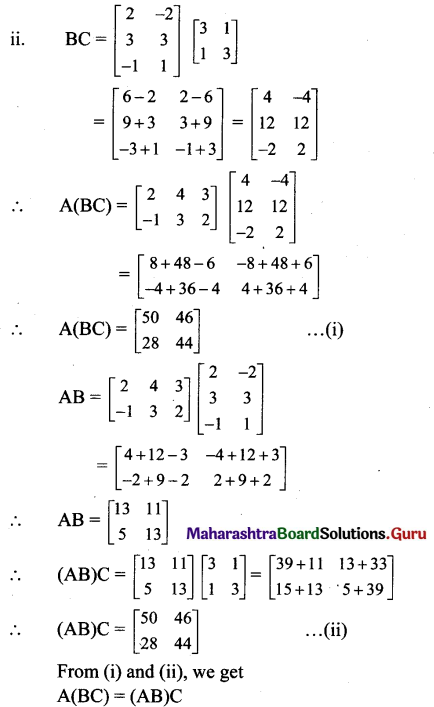Question 7.
Verify that A(B + C) = AB + AC in each of the following matrices:
i. A = $$\left[\begin{array}{cc} 4 & -2 \\ 2 & 3 \end{array}\right]$$, B = $$\left[\begin{array}{cc} -1 & 1 \\ 3 & -2 \end{array}\right]$$ and C = $$=\left[\begin{array}{cc} 4 & 1 \\ 2 & -1 \end{array}\right]$$
ii. A = $$\left[\begin{array}{ccc} 1 & -1 & 3 \\ 2 & 3 & 2 \end{array}\right]$$, B = $$\left[\begin{array}{cc} 1 & 0 \\ -2 & 3 \\ 4 & 3 \end{array}\right]$$ and C = $$\left[\begin{array}{cc} 1 & 2 \\ -2 & 0 \\ 4 & -3 \end{array}\right]$$
Solution: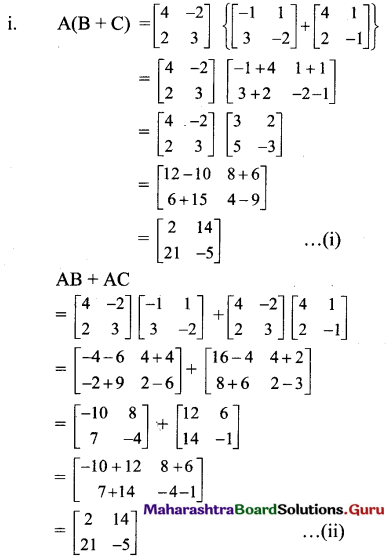From (i) and (ii), we get
A(B + C) = AB + AC.
[Note: The question has been modified.]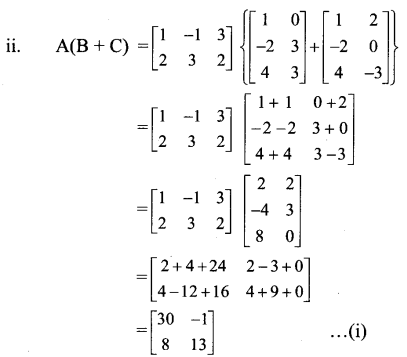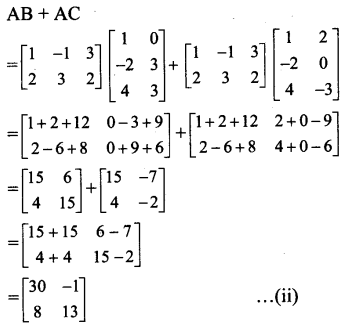Question 8.
If A = $$\left[\begin{array}{cc} 1 & -2 \\ 5 & 6 \end{array}\right]$$, B = $$\left[\begin{array}{cc} 3 & -1 \\ 3 & 7 \end{array}\right]$$, find AB – 2I, where I is unit matrix of order 2.
Solution: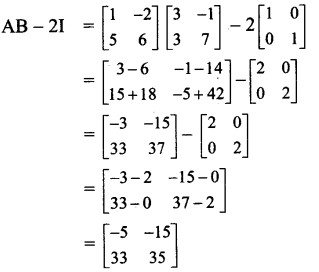Question 9.
If A = $$\left[\begin{array}{ccc} 4 & 3 & 2 \\ -1 & 2 & 0 \end{array}\right]$$, B = $$\left[\begin{array}{cc} 1 & 2 \\ -1 & 0 \\ 1 & -2 \end{array}\right]$$, show that matrix AB is non singular.
Solution:
im
∴ AB is non-singular matrix.

Question 10.
If A = , find the product (A + I)(A – I).
Solution: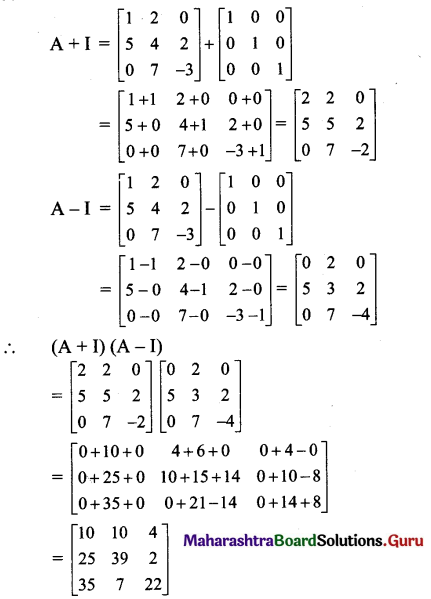[Note : Answer given in the textbook is $$\left[\begin{array}{ccc} 9 & 6 & 4 \\ 15 & 32 & -2 \\ 35 & -7 & 29 \end{array}\right]$$
However, as per our calculation it is $$\left[\begin{array}{ccc} 10 & 10 & 4 \\ 25 & 39 & 2 \\ 35 & 7 & 22 \end{array}\right]$$. ]

Question 11.
If A = $$\left[\begin{array}{ll} \alpha & 0 \\ 1 & 1 \end{array}\right]$$, B = $$\left[\begin{array}{ll} 1 & 0 \\ 2 & 1 \end{array}\right]$$, find α, if A2 = B.
Solution:
A2 = B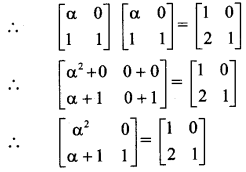∴ By equality of matrices, we get
α2 = 1 and α + 1 = 2
∴ α = ± 1 and α = 1
∴ α = 1

Question 12.
If A = $$\left[\begin{array}{lll} 1 & 2 & 2 \\ 2 & 1 & 2 \\ 2 & 2 & 1 \end{array}\right]$$, show that A2 – 4A is scalar matrix.
Solution:
A2 – 4A = A.A – 4A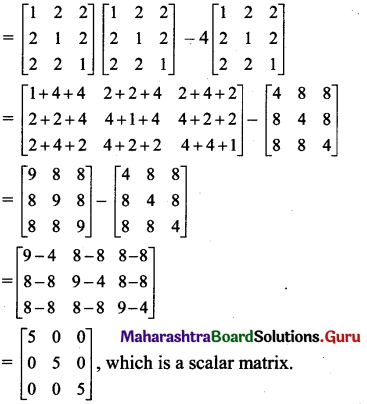Question 13.
If A = $$\left[\begin{array}{cc} 1 & 0 \\ -1 & 7 \end{array}\right]$$, find k so that A2 – 8A – kI = O, where I is a unit matrix and O is a null matrix of order 2.
Solution:
A2 – 8A – kI = O
∴ A.A – 8A – kI = O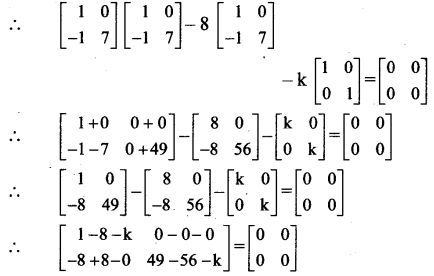∴ by equality of matrices, we get
1 – 8 – k = 0
∴ k = -7Question 14.
If A = $$\left[\begin{array}{cc} 8 & 4 \\ 10 & 5 \end{array}\right]$$, B = $$\left[\begin{array}{cc} 5 & -4 \\ 10 & -8 \end{array}\right]$$, show that (A+B)2 = A2 + AB + B2.
Solution:
We have to prove that (A + B)2 = A2 + AB + B2,
i.e., to prove A2 + AB + BA + B2 = A2 + AB + B2,
i.e., to prove BA = 0.
BA = $$\left[\begin{array}{cc} 5 & -4 \\ 10 & -8 \end{array}\right]\left[\begin{array}{cc} 8 & 4 \\ 10 & 5 \end{array}\right]$$
$$\left[\begin{array}{cc} 40-40 & 20-20 \\ 80-80 & 40-40 \end{array}\right]=\left[\begin{array}{ll} 0 & 0 \\ 0 & 0 \end{array}\right]$$

Question 15.
If A = $$\left[\begin{array}{cc} 3 & 1 \\ -1 & 2 \end{array}\right]$$, prove that A2 – 5A + 7I = 0, where I is unit matrix of order 2.
Solution:
A2 – 5A + 7I = 0 = A.A – 5A + 7I = 0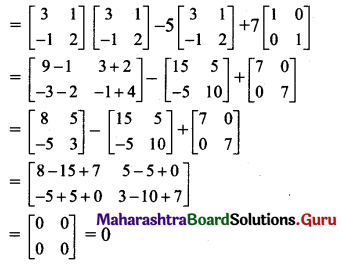Question 16.
If A = $$\left[\begin{array}{cc} 3 & 4 \\ -4 & 3 \end{array}\right]$$ and B = $$\left[\begin{array}{cc} 2 & 1 \\ -1 & 2 \end{array}\right]$$, show that (A + B)(A – B) = A2 – B2.
Solution:
We have to prove that (A + B)(A – B) = A2 – B2,
i.e., to prove A2 – AB + BA – B2 = A2 – B2,
i.e., to prove – AB + BA = 0,
i.e., to prove AB – BA.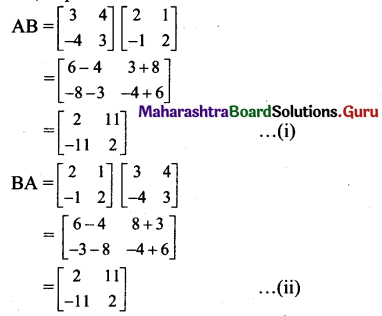From (i) and (ii), we get AB = BA

Question 17.
If A = $$\left[\begin{array}{cc} 1 & 2 \\ -1 & -2 \end{array}\right]$$, B = $$\left[\begin{array}{cc} 2 & a \\ -1 & b \end{array}\right]$$ and (A + B)2 = A2 + B2, find the values of a and b.
Solution:
Given, (A + B)2 = A2 + B2
∴ A2 + AB + BA + B2 = A2 + B2
∴ AB + BA = 0
∴ AB = -BA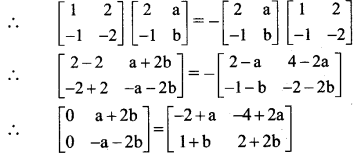∴ by equality of matrices, we get
– 2 + a = 0 and 1 + b = 0
a = 2 and b = -1
[Note: The question has been modified.]

Question 18.
Find matrix X such that AX = B,
where A = $$\left[\begin{array}{cc} 1 & -2 \\ -2 & 1 \end{array}\right]$$ and B = $$\left[\begin{array}{c} -3 \\ -1 \end{array}\right]$$
Solution:
Let X = $$\left[\begin{array}{c} a \\ b \end{array}\right]$$
But AX = B
∴ $$\left[\begin{array}{cc} 1 & -2 \\ -2 & 1 \end{array}\right]\left[\begin{array}{l} \mathrm{a} \\ \mathrm{b} \end{array}\right]=\left[\begin{array}{r} -3 \\ -1 \end{array}\right]$$
∴ $$\left[\begin{array}{c} a-2 b \\ -2 a+b \end{array}\right]=\left[\begin{array}{l} -3 \\ -1 \end{array}\right]$$
By equality of matrices, we get
a – 2b = -3 …(i)
-2a + b = -l …(ii)
By (i) x 2 + (ii), we get
-3b =-7
∴ b = $$\frac{7}{3}$$
Substituting b = $$\frac{7}{3}$$ in (i), we get
a – 2 ($$\frac{7}{3}$$) = -3
∴ a = -3 + $$\frac{14}{3}=\frac{5}{3}$$
∴ X = $$\left[\begin{array}{l} \frac{5}{3} \\ \frac{7}{3} \end{array}\right]$$Question 19.
Find k, if A = $$\left[\begin{array}{ll} 3 & -2 \\ 4 & -2 \end{array}\right]$$ and A2 = KA – 2I
Solution:
A2 = kA – 2I
∴ AA + 2I = kA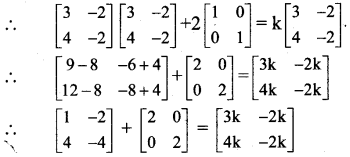∴ $$\left[\begin{array}{ll} 3 & -2 \\ 4 & -2 \end{array}\right]=\left[\begin{array}{ll} 3 k & -2 k \\ 4 k & -2 k \end{array}\right]$$
∴ By equality of matrices, we get
3k = 3
∴ k = 1

Question 20.
Find x, if $$\left[\begin{array}{lll} 1 & x & 1 \end{array}\right]\left[\begin{array}{ccc} 1 & 2 & 3 \\ 4 & 5 & 6 \\ 3 & 2 & 5 \end{array}\right]\left[\begin{array}{c} 1 \\ -2 \\ 3 \end{array}\right]$$ = 0
Solution: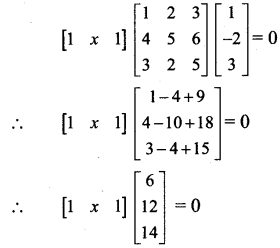∴ [6 + 12x + 14] =
∴ By equality of matrices, we get
∴ 12x + 20 = 0
∴ 12x =-20
∴ x = $$\frac{-5}{3}$$

Question 21.
Find x and y, if $$\left\{4\left[\begin{array}{ccc} 2 & -1 & 3 \\ 1 & 0 & 2 \end{array}\right]-\left[\begin{array}{ccc} 3 & -3 & 4 \\ 2 & 1 & 1 \end{array}\right]\right\}\left[\begin{array}{c} 2 \\ -1 \\ 1 \end{array}\right]=\left[\begin{array}{l} x \\ y \end{array}\right]$$
Solution: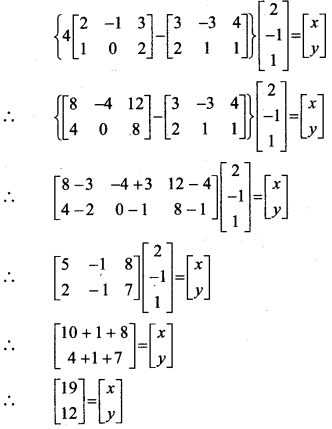∴ By equality of matrices, we get
x = 19 andy = 12Question 22.
Find x, y, z if
$$\left\{3\left[\begin{array}{ll} 2 & 0 \\ 0 & 2 \\ 2 & 2 \end{array}\right]-4\left[\begin{array}{cc} 1 & 1 \\ -1 & 2 \\ 3 & 1 \end{array}\right]\right\}\left[\begin{array}{l} 1 \\ 2 \end{array}\right]=\left[\begin{array}{c} x-3 \\ y-1 \\ 2 z \end{array}\right]$$
Solution: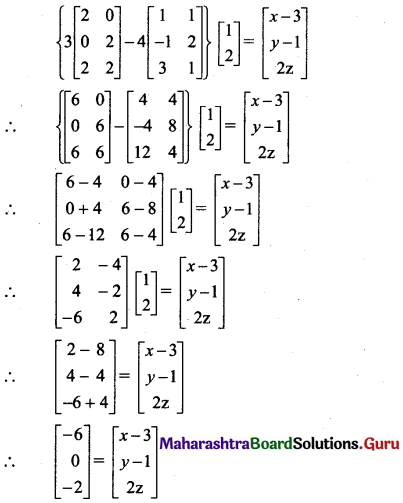∴ By equality of matrices, we get
x – 3 = -6,y – 1 = 0, 2z = -2
∴ x = – 3, y = 1, z = – 1

Question 23.
If A = $$\left[\begin{array}{cc} \cos \alpha & \sin \alpha \\ -\sin \alpha & \cos \alpha \end{array}\right]$$ show that A2 = $$=\left[\begin{array}{cc} \cos 2 \alpha & \sin 2 \alpha \\ -\sin 2 \alpha & \cos 2 \alpha \end{array}\right]$$
Solution: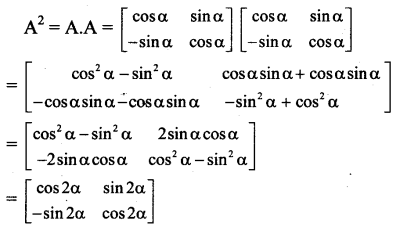Question 24.
If A = $$\left[\begin{array}{ll} 1 & 2 \\ 3 & 5 \end{array}\right]$$, B = $$\left[\begin{array}{cc} 0 & 4 \\ 2 & -1 \end{array}\right]$$
show that AB ≠ BA, but |AB| = |A| . |B|.
Solution:
AB = $$\left[\begin{array}{ll} 1 & 2 \\ 3 & 5 \end{array}\right]\left[\begin{array}{cc} 0 & 4 \\ 2 & -1 \end{array}\right]$$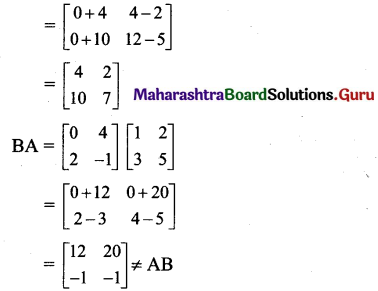Now, |AB| = $$\left|\begin{array}{cc} 4 & 2 \\ 10 & 7 \end{array}\right|$$ = 28 – 20 = 8
|A| = $$\left|\begin{array}{ll} 1 & 2 \\ 3 & 5 \end{array}\right|$$ = 5 – 6 = -1
|B| = $$\left|\begin{array}{cc} 0 & 4 \\ 2 & -1 \end{array}\right|$$ = 0 – 8 = -8
∴ |A| . |B| = (-1).(-8) = 8 = |AB|
∴ AB ≠ BA, but |AB| = |A|.|B|Question 25.
Jay and Ram are two friends in a class. Jay wanted to buy 4 pens and 8 notebooks, Ram wanted to buy 5 pens and 12 notebooks. Both of them went to a shop. The price of a pen and a notebook which they have selected was 6 and ₹ 10. Using matrix multiplication, find the amount required from each one of them.
Solution:
Let A be the matrix of pens and notebooks and B be the matrix of prices of one pen and one notebook.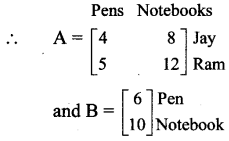The total amount required for each one of them is obtained by matrix AB.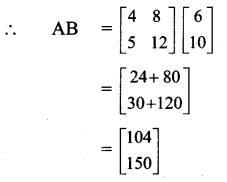∴ Jay needs ₹ 104 and Ram needs ₹ 150.